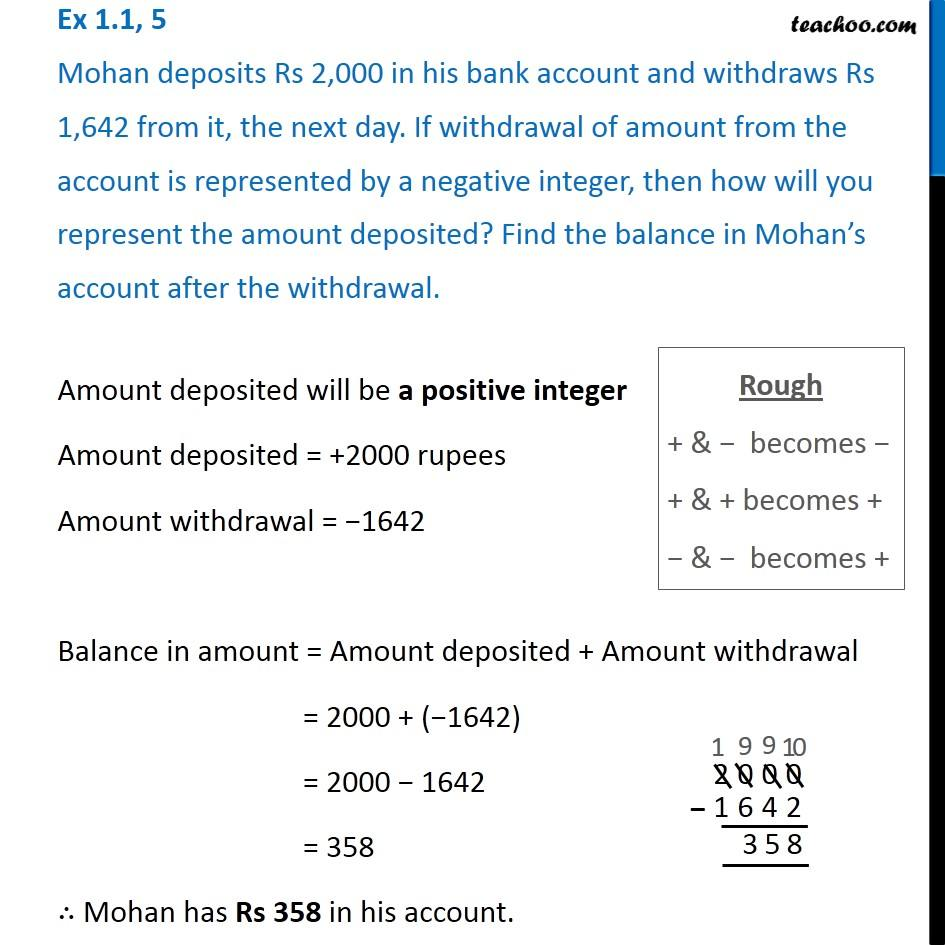1. Chapter 1 Class 7 Integers
2. Serial order wise
3. Ex 1.1

Transcript

Ex 1.1, 5 Mohan deposits Rs 2,000 in his bank account and withdraws Rs 1,642 from it, the next day. If withdrawal of amount from the account is represented by a negative integer, then how will you represent the amount deposited? Find the balance in Mohan s account after the withdrawal. Amount deposited will be a positive integer Amount deposited = +2000 rupees Amount withdrawal = 1642 Balance in amount = Amount deposited + Amount withdrawal = 2000 + ( 1642) = 2000 1642 = 358 Mohan has Rs 358 in his account. Rough + & becomes + & + becomes + & becomes +

Ex 1.1

Chapter 1 Class 7 Integers
Serial order wise